### Home > CC3 > Chapter 7 > Lesson 7.2.3 > Problem7-63

7-63.
1. For each equation below, solve for x and check your answer. Homework Help ✎

1. 10(0.02x − 0.01) = 1

2.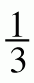x − 6 = 8

3.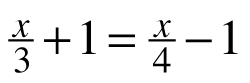4. 0.9x − 2.1 + 0.9 = 0.2(5 − x)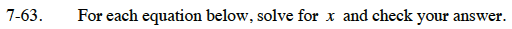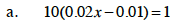Distribute and multiply by 10.
20x − 10 = 100

Add 10 to both sides.
20x = 110

Divide both sides by 20.

x = 5.5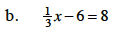Multiply by a common denominator to remove fractions. Refer to part (a) for more help.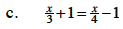Refer to part (b).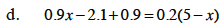Refer to part (a).

x = 2